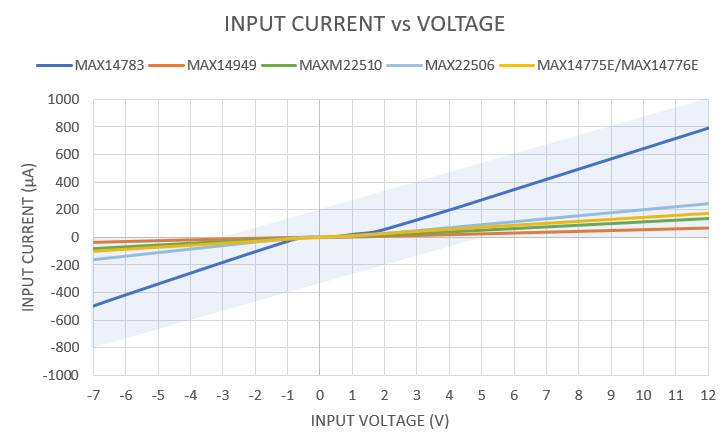# Calculating the Maximum Number of Nodes on an RS-485 Bus

### Abstract

At low data rates, the maximum number of transceivers and receivers on an RS-485 bus has a practical limit imposed by the voltage seen by receivers in relation to their input threshold voltage. The input impedance of an RS-485 receiver is not fixed in the standard and can vary between parts. This application note explains how to calculate the maximum number of receivers on a bus from data present in the data sheets for low and standard data rates.

### Introduction

The TIA/EIA-485-A standard, commonly known as RS-485, has been a staple of industrial communication for many years. The RS-485 is a standard bi-directional half-duplex or full-duplex data transmission and allows multiple receivers and drivers on a shared bus. Figure 1 shows a simplified block diagram of a simple RS-485 bus.Figure 1. Simplified RS-485 network diagram.

The RS-485 standard begins by defining a hypothetical term called the "unit load" (UL). The DC, or static, load of an RS-485 transceiver is defined by a fractional multiple of 1UL. Figure 2 shows the receiver single-ended input current-voltage mask that defines 1UL, as specified in the standard.Figure 2. Single unit load current-voltage characteristics defined in the RS-485 standard.

The RS-485 receiver measurements are expected to fall within the mask over the entire -7V to +12V span. Typical receiver input current measurements for various Maxim RS-485 transceivers are shown in Figure 3. The mask has been superimposed in the figure to highlight that the input current characteristics are within the required limits over the entire -7V to +12V input voltage span required in the RS-485 standard.Figure 3. Single unit load current-voltage characteristics for various Maxim RS-485 transceivers.

Every RS-485 transceiver or receiver can be specified with regards to the number of unit loads (nUL) that it represents on the bus.

The RS-485 standard does not explicitly declare a maximum number of nodes for an RS-485 bus and, instead, outlines the method to calculate the maximum value. Paraphrased, the calculation is as follows: the equivalent number of unit loads, nUL, that a receiver represents, is the ratio of the receiver's input current to the standard 1UL current. It is expected that the receiver input currents are usually highest at the -7V and +12V input voltages, and, as such, most manufacturers specify them at these extreme inputs. The input current ratios are calculated at these two inputs and the nUL is, then, the greater of the two ratios.

### Calculate the Maximum UL (nMAX) on a Bus

The RS-485 standard limits the maximum driver current such that a standard bus can reliably support no more than 32UL. To calculate the maximum number of nodes on a bus, divide this value by the total sum of the fractional ULs: nMAX = 32UL / (nUL1 + nUL2 + … nULn)

### Example 1: How Many MAX22506E Transceivers?

The following is an example calculation using Maxim's MAX22506E 50Mbps half-duplex transceiver. The MAX22506E datasheet specifies the receiver inputs as shown in Table 1.

Table 1. MAX22506E Receiver Input Characteristics

 Input Current (A and B) IA,B DE = GND, VCC = GND, +3.6V or +5.5V VIN = +12V +390 (max) µA VIN = -7V -360 (min)

Using this information, and the -7V and +12V current levels shown in Figure 2 (-0.8mA and +1mA, respectively), the fractional UL value at the -7V and +12V intercept points can be calculated as follows:

At the -7V intercept point: nULMAX22506E = -0.36mA/-0.8mA = 0.45UL

At the +12V intercept point: nULMAX22506E = +0.39mA/+1mA = 0.39UL

The larger of these two values, 0.45UL, is the fractional unit load of the MAX22506E.

Assuming a bus comprised of only MAX22506E simplifies the nMAX calculation to:

nMAX = 32UL/0.45UL

nMAX = 71 MAX22506E transceivers can comprise a single RS-485 bus.

### Example 2: How Many Transceivers on a Mixed Bus?

The following is an example calculation using three different transceivers on a single RS-485 bus. This example uses Maxim's MAX22506E, the MAX14783E, and the fault protected MAX14776E. All of these transceivers operate at 3.3V to 5V, with a maximum data rate of at least 20Mbps, and all are half-duplex. The receiver input characteristics for each of these is shown in Figure 3.

Table 2 shows the receiver input characteristics for the 3 devices, as taken from their respective datasheets.

Table 2. Receiver Input Current Specifications

Input Voltage
(A and B)
Maximum Input Current (µA)
MAX22506E MAX14783E MAX14776E
VIN = -7V -360 -800 -200
VIN = +12V +390 +1000 +280

Using the information in this table, the fractional UL value at the -7V and +12V intercept points can be calculated as shown in Table 3.

Table 3. Fractional Unit Load Calculations

Input voltage
(A and B)

nUL Calculation
for Transceivers

MAX22506E MAX14783E MAX14776E
VIN = -7V -0.36mA/-0.8mA = 0.45UL -0.8mA/-0.8mA = 1UL -0.20mA/-0.8mA = 0.25UL
VIN = +12V 0.39mA/1mA = 0.39UL +1.0mA/1mA = 1UL +0.28mA/1mA = 0.28UL

The larger of the two ratios, for each device, is the fractional UL. As shown in the table, this is 0.45UL for the MAX22506E, 1UL for the MAX14783E, and 0.28UL for the MAX14776E.

The maximum number of transceivers on the bus is calculated as follows:

nMAX = 32UL / (nUL1 + nUL2 + … nULn)

Using the calculated values, Table 4 shows some of the valid combinations (32 UL, max) of these transceivers that can be used on a single RS-485 bus.

Table 4. Mixed RS-485 Bus Nodes

MAX22506E

MAX14783E

MAX14776E

Total UL

Transceivers UL Transceivers UL Transceivers UL
1 0.45 30 30 5 1.4 31.9
10 4.5 25 25 8 2.2 31.7
12 5.4 20 20 23 6.4 31.8
16 7.2 12 12 45 12.6 31.8
20 9 18 18 17 4.8 31.8
25 11.3 15 15 20 5.6 31.9
45 20.3 9 9 9 2.5 31.8